# Program to check Evil Number In Java

In this tutorial you will learn about the Program to check Evil Number In Java and its application with practical example.

In this tutorial, we will learn to create a Java Program to check Evil Numbers in Java using Java programming.

## Prerequisites

Before starting with this tutorial we assume that you are best aware of the following Java programming topics:

• Java Operators.
• Basic Input and Output function in Java.
• Class and Object in Java.
• Basic Java programming.
• If-else statements in Java.
• For loop in Java.

## What is Evil Number?

So a Number called an Evil number if it has an even number of 1’s and they are binary equivalent i.e in pair of 2,4 and so on…

Example: 15 is a Evil number because it has Binary Equivalent of 4 ones: 1111.

## Program to check Evil Number In Java

In this  program we will find given number is a Evil number or not in using java programming.We would first declared and initialized the required variables. Next, we would prompt user to input the a number.Later we  find number is Evil number or not let’s have a look at the code.

## Output

Evil Number.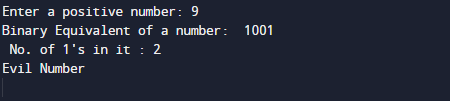Not a Evil Number.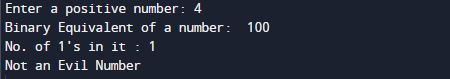In the above program, we have first declared and initialized a set variables required in the program.

• number= it will hold entered number.
• pow= it will provide power of 2 in binary.
• bin= it will also hold binary result.
• c= it will count 1 in binary value.

After declaring variables in the next statement user will be prompted to enter a value and  which will be assigned to variable ‘number’.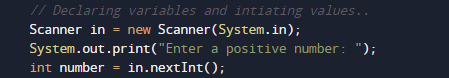And after that we will find binary of a given number and number of one in binary digit of a number as shown in picture below.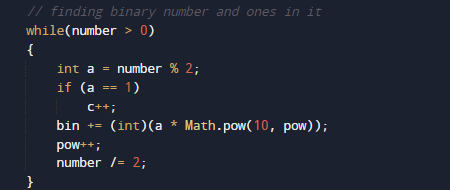then we will print the Binary value of number and number of one in it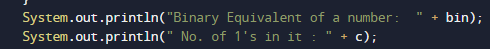And finally we will check that if number of 1’s in it are present in even or odd.if even number is Evil if not number is not a evil number.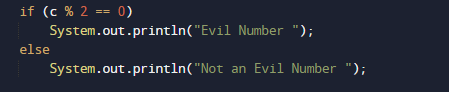In this tutorial we have learn about the Program to check Evil Number In Java and its application with practical example. I hope you will like this tutorial.